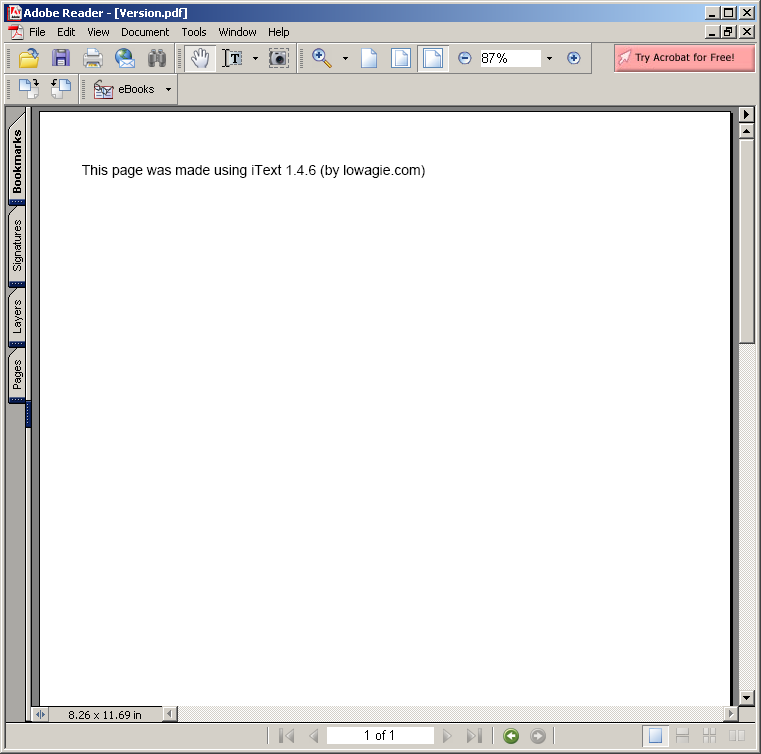# Utilities for flop (floating-point operation) counting. : Algorithms « Collections Data Structure « Java

Utilities for flop (floating-point operation) counting.

```

/* Copyright (C) 2006 Univ. of Massachusetts Amherst, Computer Science Dept.
This file is part of "MALLET" (MAchine Learning for LanguagE Toolkit).
http://www.cs.umass.edu/~mccallum/mallet
This software is provided under the terms of the Common Public License,
version 1.0, as published by http://www.opensource.org.  For further
information, see the file `LICENSE' included with this distribution. */
//package cc.mallet.grmm.util;

/**
* Utilities for flop (floating-point operation) counting.  This is a much better
*  way to measure computation than CPU time, because it avoids measuring non-essential
*  properties of the implementations.
*
* \$Id: Flops.java,v 1.1 2007/10/22 21:37:58 mccallum Exp \$
*/
public class Flops {

private static long flops = 0;

// this figures taken from Tom Minka's lightspeed
private static final int EXP_FLOPS = 40;
private static final int LOG_FLOPS = 20;
private static final int DIV_FLOPS = 8;
private static final int SQRT_FLOPS = 8;

public static long getFlops ()
{
return flops;
}

public static void exp ()
{
flops += EXP_FLOPS;
}

public static void log ()
{
flops += LOG_FLOPS;
}

public static void div ()
{
flops += DIV_FLOPS;
}

public static void sqrt ()
{
flops += SQRT_FLOPS;
}

public static void sumLogProb (int n)
{
flops += n * (LOG_FLOPS + EXP_FLOPS);
}

public static void increment (int N)
{
flops += N;
}

public static void log (int N)
{
flops += N * LOG_FLOPS;
}

public static void exp (int N)
{
flops += N * EXP_FLOPS;
}

public static void pow (int N)
{
// Get an upper bound using
//    a^b = exp(b*log(a))
Flops.increment (N * (EXP_FLOPS + LOG_FLOPS + 1));
}

public static class Watch {

private long starting;
private long current;

public Watch ()
{
starting = flops;
current = starting;
}

public long tick ()
{
return tick (null);
}

public long tick (String message)
{
long elapsed = flops - current;
current = flops;
if (message != null) System.err.println (message+" flops = "+elapsed);
return elapsed;
}

public long totalFlopsElapsed () { return flops - starting; }
}
}

```

### Related examples in the same category

 1 Anagrams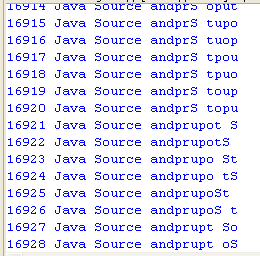2 Hanoi puzzle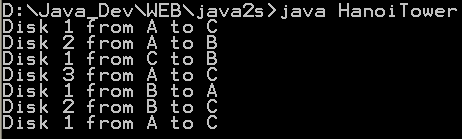3 Fibonacci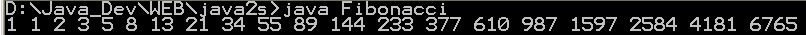4 Sieve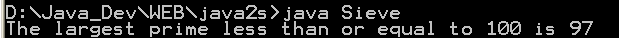5 Find connections using a depth-first search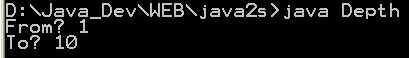6 Find connections using hill climbing. 7 Find optimal solution using least-cost 8 Find the lost keys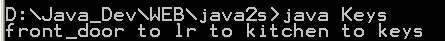9 Compute the area of a triangle using Heron's Formula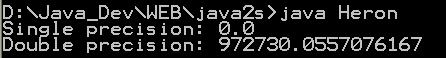10 Compute prime numbers 11 Print a table of fahrenheit and celsius temperatures 1 12 Print a table of fahrenheit and celsius temperatures 2 13 Print a table of Fahrenheit and Celsius temperatures 3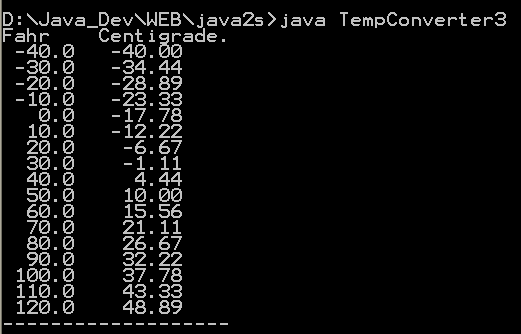14 Soundex - the Soundex Algorithm, as described by Knuth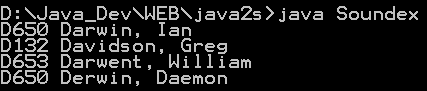15 A programmable Finite State Machine implementation. 16 An extendable Graph datastructure. 17 LU Decomposition 18 Reverse Polish Notation 19 Permutator test 20 implements the LZF lossless data compression algorithm 21 Linear Interpolation 22 Utility class for generating the k-subsets of the numbers 0 to n 23 Version